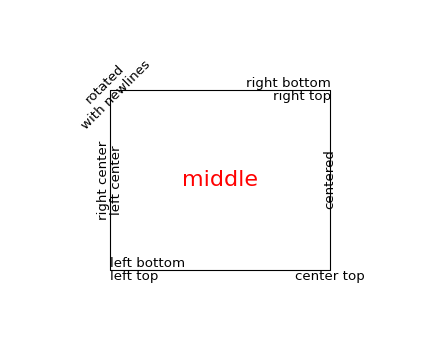#### Previous topic

Text introduction

#### Next topic

Writing mathematical expressions

# Text properties and layout¶

The matplotlib.text.Text instances have a variety of properties which can be configured via keyword arguments to the text commands (eg title(), xlabel() and text()).

Property Value Type
alpha float
backgroundcolor any matplotlib color
bbox rectangle prop dict plus key ‘pad’ which is a pad in points
clip_box a matplotlib.transform.Bbox instance
clip_on [True | False]
clip_path a Path instance and a Transform instance, a Patch
color any matplotlib color
family [ ‘serif’ | ‘sans-serif’ | ‘cursive’ | ‘fantasy’ | ‘monospace’ ]
fontproperties a matplotlib.font_manager.FontProperties instance
horizontalalignment or ha [ ‘center’ | ‘right’ | ‘left’ ]
label any string
linespacing float
multialignment [‘left’ | ‘right’ | ‘center’ ]
name or fontname string eg, [‘Sans’ | ‘Courier’ | ‘Helvetica’ ...]
picker [None|float|boolean|callable]
position (x,y)
rotation [ angle in degrees ‘vertical’ | ‘horizontal’
size or fontsize [ size in points | relative size eg ‘smaller’, ‘x-large’ ]
style or fontstyle [ ‘normal’ | ‘italic’ | ‘oblique’]
text string or anything printable with ‘%s’ conversion
transform a matplotlib.transform transformation instance
variant [ ‘normal’ | ‘small-caps’ ]
verticalalignment or va [ ‘center’ | ‘top’ | ‘bottom’ | ‘baseline’ ]
visible [True | False]
weight or fontweight [ ‘normal’ | ‘bold’ | ‘heavy’ | ‘light’ | ‘ultrabold’ | ‘ultralight’]
x float
y float
zorder any number

You can layout text with the alignment arguments horizontalalignment, verticalalignment, and multialignment. horizontalalignment controls whether the x positional argument for the text indicates the left, center or right side of the text bounding box. verticalalignment controls whether the y positional argument for the text indicates the bottom, center or top side of the text bounding box. multialignment, for newline separated strings only, controls whether the different lines are left, center or right justified. Here is an example which uses the text() command to show the various alignment possibilities. The use of transform=ax.transAxes throughout the code indicates that the coordinates are given relative to the axes bounding box, with 0,0 being the lower left of the axes and 1,1 the upper right.

```import matplotlib.pyplot as plt
import matplotlib.patches as patches

# build a rectangle in axes coords
left, width = .25, .5
bottom, height = .25, .5
right = left + width
top = bottom + height

fig = plt.figure()

# axes coordinates are 0,0 is bottom left and 1,1 is upper right
p = patches.Rectangle(
(left, bottom), width, height,
fill=False, transform=ax.transAxes, clip_on=False
)

ax.text(left, bottom, 'left top',
horizontalalignment='left',
verticalalignment='top',
transform=ax.transAxes)

ax.text(left, bottom, 'left bottom',
horizontalalignment='left',
verticalalignment='bottom',
transform=ax.transAxes)

ax.text(right, top, 'right bottom',
horizontalalignment='right',
verticalalignment='bottom',
transform=ax.transAxes)

ax.text(right, top, 'right top',
horizontalalignment='right',
verticalalignment='top',
transform=ax.transAxes)

ax.text(right, bottom, 'center top',
horizontalalignment='center',
verticalalignment='top',
transform=ax.transAxes)

ax.text(left, 0.5*(bottom+top), 'right center',
horizontalalignment='right',
verticalalignment='center',
rotation='vertical',
transform=ax.transAxes)

ax.text(left, 0.5*(bottom+top), 'left center',
horizontalalignment='left',
verticalalignment='center',
rotation='vertical',
transform=ax.transAxes)

ax.text(0.5*(left+right), 0.5*(bottom+top), 'middle',
horizontalalignment='center',
verticalalignment='center',
fontsize=20, color='red',
transform=ax.transAxes)

ax.text(right, 0.5*(bottom+top), 'centered',
horizontalalignment='center',
verticalalignment='center',
rotation='vertical',
transform=ax.transAxes)

ax.text(left, top, 'rotated\nwith newlines',
horizontalalignment='center',
verticalalignment='center',
rotation=45,
transform=ax.transAxes)

ax.set_axis_off()
plt.show()
```